mscroggs.co.uk
mscroggs.co.uksubscribe

# Blog

2016-06-29
In this blog post, I will present two conditions that cause a braid to fail, with reasons why. You can read more about the method of braiding we are talking about here, in part one.
In this post, we will consider the ($$a$$,$$b$$) braid with $$a$$ slits, taking the $$b$$th thread each time.

### Some strands are never moved

If some of the strads are never moved in the braiding process, then the braid will fail. To find out when this happens, I will draw lines to show the moves that are made. For the (8,3) braid, the first move is:
Then the second move is:
And so on until we get:
For the (8,3) braid, every strand is moved at some point in the cycle.
For the (6,2) braid, however, we get the following diagram:
Now some strands are not moved and the braid will fail.
To find out when all the slits are visited, I first label the slots: the slot which starts empty is 0, then I continue numbering anti-clockwise. This numbering puts all the multiples of $$a$$ at the bottom slot.
Now let's look at which slots we visit. We start at 0, then visit $$b$$, then $$2b$$, then $$3b$$ and so on. We visit all the multiples of $$b$$.
Therefore we will reach the bottom slot again and finish our loop when we reach a common multiple of $$a$$ and $$b$$. The first time this happens will be at the lowest common multiple, or $$\text{lcm}(a,b)$$.
On the way to this slot, I visited one slot for every $$a$$ we passed, so the number of slots I have visited is:
$$\frac{\text{lcm}(a,b)}{a}$$
I will visit every slot if:
$$\frac{\text{lcm}(a,b)}{a}=b$$
Or, in other words, if $$\text{lcm}(a,b)=ab$$. This is true when, $$a$$ and $$b$$ have no common factors, or are coprime: often written $$\text{hcf}(a,b)=1$$.
Therefore, if $$a$$ and $$b$$ are not coprime, then the braid sill fail.

### The braid results in twists

Some braids, for example (13,3), result in a groups of twisted strands rather than a whole braid.
To see when this happens, imagine the ($$a$$,$$b$$) braid just after a thread has been moved. Call the currently empty slit 0. The threads at positions $$a-1$$, $$a-2$$, ..., $$a-b+1$$ have just been jumped over. If, from these threads, the thread at $$a-1$$ is moved before the others, then this group of threads will form into a twist.
This will happen when $$b$$ is a factor of $$a-1$$, as this leads to $$a-1$$ being reached before slit 0 is passed again. Therefore is $$b$$ is a factor of $$a-1$$, the braid will not work.

### A conjecture

In this post, I have shown that if $$a$$ and $$b$$ are not coprime or if $$b$$ is a factor of $$a-1$$, then the braid will fail. From the examples I have tried (I'm compiling them here), it seems that all other braids will work. It seems difficult, however, to explain why all other braids work. I'd love to hear your ideas on how this could be shown.

### What's next?

A have a few leads to follow up on, which may lead to a proof or counterexample. Vicky Neale has found instructions for making Kumihimo braids. They are created in a very similar way to my cardboard braids, so looking into the patterns you can make with them may be helpful.
@mathforge has found a mathoverflow page where quotients of the braid group are discussed. I know from past discussions that the braiding group is not exactly what I'm looking for—for example, the multiple twists is considered a member of the braiding group. However, finding the correct way to adapt the group may solve the problem. Looks like I may have to polish up on my group theory...

Until a proof of or counterexample to the conjecture turns up, we seem to be at the end of the braiding puzzle. But do not fret, there is a related problem that we can now spend some time on.
In the braids we've done so far, we have taken the same jump every time. But more complicated rules could be used: for example, we could alternate between taking the third thread and the second thread. I'm yet to find a rule with different jumps that works, so let me know if you find one before me!

### Similar postsBraiding, pt. 1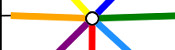Electromagnetic Field talk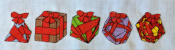Christmas cross stitch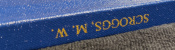PhD thesis, chapter ∞

Comments in green were written by me. Comments in blue were not written by me.

Allowed HTML tags: <br> <a> <small> <b> <i> <s> <sup> <sub> <u> <spoiler> <ul> <ol> <li>
To prove you are not a spam bot, please type "theorem" in the box below (case sensitive):
2016-06-29
Since Electromagnetic Field 2014, I have been slowly making progress on a recreational math problem about braiding. In this blog post, I will show you the type of braid I am interested in and present the problem.

### Making an (8,3) braid

To make what I will later refer to as an (8,3) braid, you will need:
• 7 lengths of coloured wool, approx 50cm each
• Cardboard
• Scissors
• A pencil
First, cut an octagon from the cardboard. The easiest way to do this is to start with a rectangle, then cut its corners off.
Next, use the pencil to punch a hole in the middle of your octagon and cut a small slit in each face of the octagon.
Now, tie the ends of your wool together, and put them through the hole. pull each strand of wool into one of the slits.
Now you are ready to make a braid. Starting from the empty slit, count around to the third strand of will. Pull this out of its slit then into the empty slit. Then repeat this starting at the newly empty slit each time. After a short time, a braid should form through the hole in the cardboard.
I call the braid you have just made the (8,3) braid, as there are 8 slits and you move the 3rd strand each time. After I first made on of these braid, I began to wonder what was special about 8 and 3 to make this braid work, and for what other numbers $$a$$ and $$b$$ the ($$a$$,$$b$$) would work.
In my next blog post, I will give two conditions on $$a$$ and $$b$$ that cause the braid to fail. Before you read that, I recommend having a go at the problem yourself. To help you on your way, I am compiling a list of braids that are known to work or fail at mscroggs.co.uk/braiding. Good luck!

### Similar postsElectromagnetic Field talk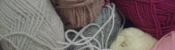Braiding, pt. 2Christmas cross stitch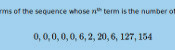Logical contradictions

Comments in green were written by me. Comments in blue were not written by me.

Allowed HTML tags: <br> <a> <small> <b> <i> <s> <sup> <sub> <u> <spoiler> <ul> <ol> <li>
To prove you are not a spam bot, please type "s" then "e" then "g" then "m" then "e" then "n" then "t" in the box below (case sensitive):
2014-09-04
Last weekend, I attended Electromagnetic Field, a camp for hackers, geeks, makers and the interested. On the Sunday, I gave a talk on four mathematical ideas/tasks which I have encountered over the past few years: Flexagons, Folding Tube Maps, Braiding and Sine Curves. I'd love to see photos, hear stories, etc from anyone who tries these activities: either comment on here or tweet @mscroggs.

### Flexagons

It's probably best to start by showing you what a flexagon is...
What you saw there is called a trihexaflexagon. Tri- because it has three faces; -hexa- because it is a hexagon; and -flexagon because it can be flexed to reveal the other faces.
The story goes that, in 1939, Arthur H. Stone, who was an Englishman studying mathematics at Princeton, was trimming the edges off his American paper to fit in his English folder. He was fiddling with the offcuts and found that if he folded the paper under itself in a loop, he could make a hexagon; and when this hexagon was folded up as we saw, it would open out to reveal a different face.
The way it flexes can be shown on a diagram: In the circles, the colour on either side of the flexagon is shown and the lines show flexes which can be made.
When Stone showed his flexagon to other students at Harvard, they were equally amazed by it, and they formed what they called 'The Flexagon Committee'. Members of the committee included Richard Feynman, who was then still a graduate student. The committee could meet regularly and soon discovered other flexagons, the first of which was the hexahexaflexagon: Again shaped like a hexagon, but this time with six faces.
A hexahexaflexagon is created by taking a longer strip of paper and rolling it around itself like this. The shorter strip at the end is then folded and glued in the same way the trihexaflexagon was. Once made, the hexahexaflexagon can be flexed. From some positions, the flexagon can be flexed in different ways to reveal different faces. Due to this, finding some of the faces can be quite difficult.
The committee went on to find other flexagons which could be made, again made by first folding into a shorter strip, then folding up like the trihexaflexagon.
The committee later found that hexaflexagons with any number of faces could be made by starting with a certain shaped strip, rolling it up then folding it like a trihexaflexagon.

An excellent article by Martin Gardner on flexagons can be found in this book.
Trihexaflexagon templates (click to enlarge then print):
Our second story starts with me sitting on the tube reading Alex's Adventures in Numberland by Alex Bellos on the tube. In his book, Alex describes how to fold a tetrahedron, or triangle-based pyramid, from two business cards. With no business cards to hand, I picked up two tube maps and followed the steps: first, I folded it corner to corner; then I folded the overlaps over.I made another one of these, but the second a mirror image of the first, slotted them together and I had my tetrahedron.
Then I made a tube map cube by making six squares like so and slotting them together.
While making these shapes, I discovered an advantage of tube maps over business cards: Due to the pages, folded tube maps have slots to tuck the tabs into, so the solids are pretty sturdy.
Making these shapes got me wondering: what other Platonic solids could I make?
In 2D, we have regular shapes: shapes with all the sides of the same length and all angles equal. Platonic solids are sort of the 3D equivalent of this: they are 3D shapes where every face is the same regular shape and at each vertex the same number of faces meet.
For example, our tetrahedron is a Platonic solid because every side is a regular triangle, and three triangles meet at every vertex. Our cube is a Platonic solid because every side is a square (which is a regular shape) and three squares meet at every vertex.
In order to fold all the Platonic solids, we must first find out how many there are.
To do this, we're going to start with a triangle, as it is the 2D shape with the smallest number of sides, and make Platonic solids.
If we try to put two triangles at each vertex, then they'll squash flat; so that's no good. We've seen that three triangles at each vertex makes a tetrahedron. If we put four triangles at each vertex then we get an octahedron.
Five triangles at each vertex gives us an icosahedron.
Each angles in an equilateral triangle is 60°. So if we put six triangles at each vertex the angles add up to 360°, a full turn. This means that the triangles will lie flat, giving us a nice pattern for a kitchen floor, but not a solid. Any more than 6 triangles will add up to more than 360 and also not give a solid. So we have found all the Platonic solids whose faces are triangles.
Next, four sided faces. Three squares at each vertex gives us a cube. Four squares at each vertex will add up to 4 times 90°... 360° again, so another kitchen floor and as before we have all the Platonic solids whose faces are squares.
Now moving up again to five sided faces. Three pentagons at each vertex will gives us a dodecahedron, which looks like this.
This is the best I could do.
(After the talk, I was shown a few better ways to fold pentagons. Watch this space for my attempts...) Now if we try four pentagons around a vertex: the internal angle in a pentagon is 108°. 4 times 108° is 432°. This is more than a full turn, so we don't get a solid.
Moving up again, if we take three hexagons we get another tessellation. Shapes with more than six sides will all have larger angles than this so three make more than a full turn. Therefore, we have found and folded all the Platonic solids.
In 2012, I posted this on my blog and got the following comment:
I'm pretty sure this was a joke, but one hour, 48 tube maps and a lot of glue later:Alex's Adventures in Numberland by Alex Bellos introduced business card folding and takes it further, finishing with a business card Menger sponge.

### Braiding

A few months ago, my mother showed my a way to make braids using a cardboard octagon with a slot cut on each side and a hole in the middle.
To make a braid, seven strands of wool are tied together, fed through the hole, then one tucked into each slot.
Now, we jump over two strands, pick the third strand and move it to the vacant slot. So first, we jump over the orange and green and move the red strand.
Then we jump the light blue and yellow and move the dark blue.
And so on..
After a while, the braid looks like this:
Once I'd made a few braids, I began to wonder which other numbers of threads could be used to make braids like this. To investigate this I found it useful to represent braids by drawing connections to show where a thread is moved. This shows the first move:
Then the second move:
And so on until you get:
After the octagon, I tried braiding on a hexagon, moving the second thread each time. Here's what happened:
I only moved the yellow and green threads and nothing interesting happened. When I drew this out as before, it demonstrated what had gone wrong: three slots are missed so three threads are never moved.
So we need to find out when slots are missed and when all the slots are hit. To do this, let's call the number of slots $$a$$, and let $$b$$ be the number thread we pick each time. For example, in the first braid that worked $$a$$ was 8 and $$b$$ was 3.
First we'll label the slots. Label the slot which starts empty 0, then number anti-clockwise. This numbering puts all the multiples of $$a$$ at the bottom slot.
Now let's look at which slots we visit. We start at0, then visit $$b$$, then $$2b$$, then $$3b$$ and so on. We visit all the multiples of $$b$$.
Therefore we will reach the bottom slot again and finish our loop when we reach a common multiple of $$a$$ and $$b$$. The first time this happens will be at the lowest common multiple, or:
$$\mbox{lcm}(a,b)$$
On our way to this slot, we visited one slot for every $$a$$ we passed, so the number of slots we have visited is
$$\frac{\mbox{lcm}(a,b)}{a}$$
and we will visit every slot if
$$\frac{\mbox{lcm}(a,b)}{a}=b$$
or, equivalently if
$$\mbox{lcm}(a,b)=ab.$$
This is true when, $$a$$ and $$b$$ have no common factors, or in other words are coprime; which can be written
$$\mbox{hcf}(a,b)=1.$$
So we've found that if $$a$$ is the number of slots and $$b$$ is the jump then the braid will not work unless $$a$$ and $$b$$ are coprime.
For example, if $$a$$ is 6 and $$b$$ is 2 then 2 is a common factor so the braid fails. And, if $$a$$ is 8 and $$b$$ is 3 then there are no common factors and the braid works. And, if $$a$$ is 12 and $$b$$ is 5 then there are no common factors and the braid works.
But, if $$a$$ is 5 and $$b$$ is 2 then there are no common factors but the braid fails.
The rule I've explained is still correct, and explains why some braids fail. But if $$a$$ and $$b$$ are coprime, we need more rules to decide whether or not the braid works.
And that's as far as I've got, so I'm going to finish braiding with two open questions: Why does the 5 and 2 braid fail? And for which numbers $$a$$ and $$b$$ does the braid work?

### Sine curves

For the last part of the talk, I did a practical demonstration of how to draw a sine curve using five people.
I told the first person to stand on the spot and the second person to stand one step away, hold a length of string and walk.
The third person was instructed to stay in line with the second person, while staying on a vertical line.
The fourth person was told to walk in a straight line at a constant speed.
And the fifth person had to stay in line with both the third and fourth people. This led them to trace a sine curve.
To explain why this is a sine curve, consider the following triangle:
As our first two people are one step apart, the hypotenuse of this triangle is 1. And so the opposite (vertical) side is equal to the sine of the angle.
I like to finish with a challenge, and this task leads nicely into two challenge questions:
1. How could you draw a cosine curve with five people?
2. How could you draw a tan(gent) curve with five people?

People Maths: Hidden Depths is full of this kind of dynamic task involving moving people.

### Similar postsTube map Platonic solids, pt. 3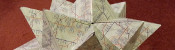Tube map Platonic solids, pt. 2Tube map kaleidocyclesBraiding, pt. 1

Comments in green were written by me. Comments in blue were not written by me.